# MMIC Phase Shifter Example 1

Here's something that you won't see on the TriQuint web site, the RMS phase error calculation with actual phase state data. This data was contributed by AMTL, thanks guys! Here we'll plug in some real data to the equations we outlined on this page and this page.

This phase shifter operates at S-band, and has six bits. Therefore it has 64 phase states, and the smallest bit is ~ 6 degrees (5.625 if you want to be exact!) Here's the raw phase states. We plotted the "reference state" in dark line so you can see that it is nothing special.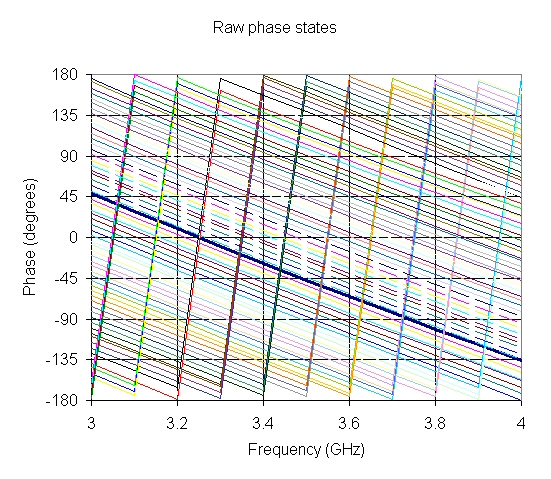Here's the raw relative phase: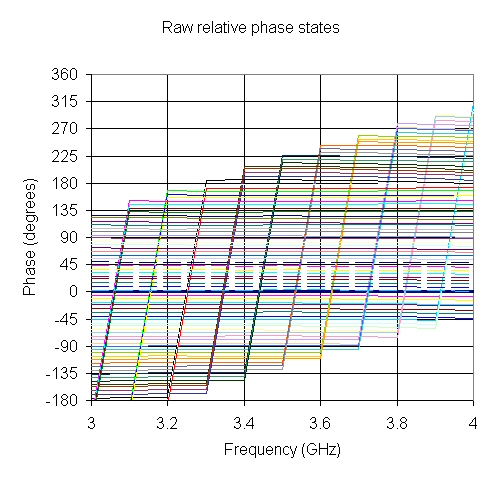Here's what you get when you plug in that "modulo" function: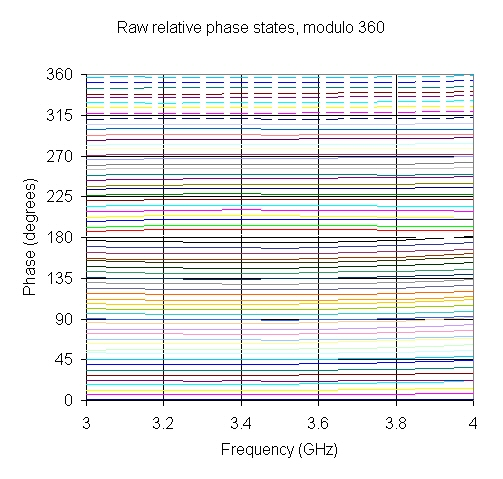Here's the raw phase errors: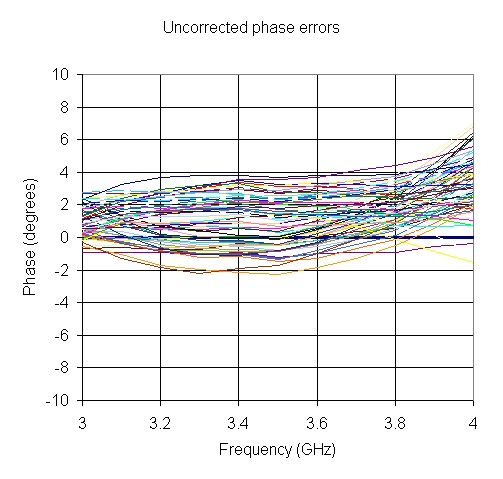Here's the corrected phase errors: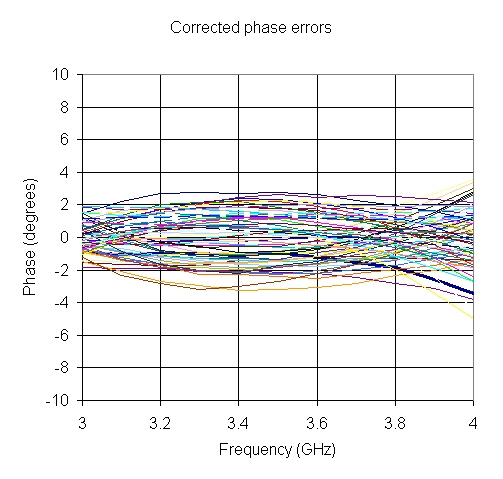Here's the RMS errors: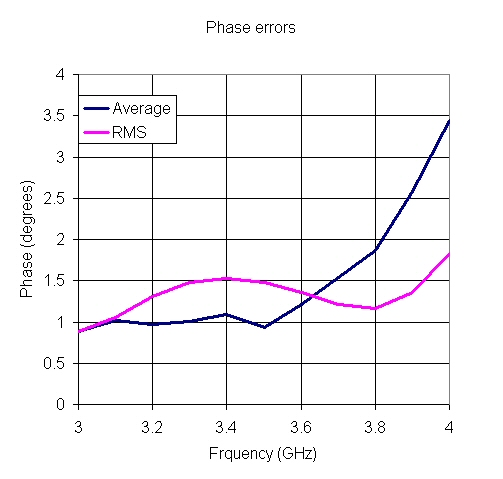Author : Unknown Editor